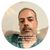Announcements
MAINTENANCE ALERT: Dec. 12th starting 9 AM CET. Community will be read-only. GET DETAILS
cancel
Showing results for
Did you mean:Not applicable

## How to get positive values and negative values separated?

Hi All,

The below table box is the output I am getting

The average value is the output based on date and value

 Name Date Value Avg.Value. A 1/1/2017PM 4 0 B 1/2/2017PM 7 0.75 C 1/3/2017PM 4 -0.42 D 1/4/2017PM 3 -0.25

The expression to get average value based on below formula:

Num((Sum(Rolling_7_days.Counts)-above(TOTAL sum( Rolling_7_days.Counts ) ))/above(TOTAL sum( Rolling_7_days.Counts ) ),'#,##0.00')

According to the Formula.

(Today-Previous day)/Previous day)*100)/Previous day)*100)/Previous day)*100)/Previous day)*100)/Previous day)*100)/Previous day)*100

Name B:

Today=1/2/2017                value:7:7

Previous day=1/1/2017      value:  4

Formula:

(7-4)/4=0.75

Name C:

Today=1/3/2017                value:4:4:4

Previous day=1/2/2017      value:  7

Formula:

(4-7)/7=-0.42

NameD:

Today=1/4/2017                value:3:3:3

Previous day=1/3/2017      value:4:4:4

Formula:

(3-4)/4=-0.25

so I need a separate table box for positive values and separate table box for negative values based on above expresson

Thanks,

Muthu

1 Solution

Accepted SolutionsSpecialist

This:

if(

(Sum(Rolling_7_days.Counts)-above(TOTAL sum( Rolling_7_days.Counts ) ))/above(TOTAL sum( Rolling_7_days.Counts ) )>=0,

(Sum(Rolling_7_days.Counts)-above(TOTAL sum( Rolling_7_days.Counts ) ))/above(TOTAL sum( Rolling_7_days.Counts ) ))

is not working?

10 RepliesMVP

Can you share expected output, and from table box do you mean straight table? so you want one straight table with positive values and another one with negative values?, more info will help. ThankNot applicable
Author

Yes I want separate Straight table for Positive values and separate Straight table for Negative values

i want the output like

Positive Straight table :

 Name Date Value Avg.Value. A 1/1/2017PM 4 0 B 1/2/2017PM 7 0.75

Negative Straight table:

 Name Date Value Avg.Value. C 1/3/2017PM 4 -0.42 D 1/4/2017PM 3 -0.25

Thanks,

MuthuSpecialist

Hello, Mathu!

Why you don't wonna use IF condition?

If(formula_result>=0,formula result) for posivite table, and <0 for negative table.Specialist II

‌for positive values use range Max function as

rangemax(yourexpression,0)

for negative values use range min function as

rangemin(yourexpression,0)Not applicable
Author

The expression to get average value based on below formula:

Num((Sum(Rolling_7_days.Counts)-above(TOTAL sum( Rolling_7_days.Counts ) ))/above(TOTAL sum( Rolling_7_days.Counts ) ),'#,##0.00')

Thanks,

MuthuSpecialist

This:

if(

(Sum(Rolling_7_days.Counts)-above(TOTAL sum( Rolling_7_days.Counts ) ))/above(TOTAL sum( Rolling_7_days.Counts ) )>=0,

(Sum(Rolling_7_days.Counts)-above(TOTAL sum( Rolling_7_days.Counts ) ))/above(TOTAL sum( Rolling_7_days.Counts ) ))

is not working?Champion

for Negative values

If(Num((Sum(Rolling_7_days.Counts)-above(TOTAL sum( Rolling_7_days.Counts ) ))/above(TOTAL sum( Rolling_7_days.Counts ) ),'#,##0.00')<0,Num((Sum(Rolling_7_days.Counts)-above(TOTAL sum( Rolling_7_days.Counts ) ))/above(TOTAL sum( Rolling_7_days.Counts ) ),'#,##0.00'))

for positive values

If(Num((Sum(Rolling_7_days.Counts)-above(TOTAL sum( Rolling_7_days.Counts ) ))/above(TOTAL sum( Rolling_7_days.Counts ) ),'#,##0.00')>=0,Num((Sum(Rolling_7_days.Counts)-above(TOTAL sum( Rolling_7_days.Counts ) ))/above(TOTAL sum( Rolling_7_days.Counts ) ),'#,##0.00'))

Sunil ChauhanNot applicable
Author

Yes it is not working.

based on my requirement my question is wrong

But the answer what you said is correct

thanks for your Expression now i will close this discussion by marking your answer as correct will open a new discussion with the correct requirement. Please Reply in that

Thanks,

MuthuMVP

Would you able to share sample

Before develop something, think If placed (The Right information | To the right people | At the Right time | In the Right place | With the Right context)Community Browser